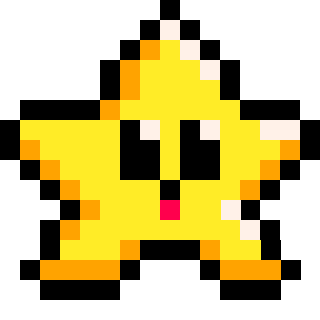# TONUM

``tonum( a )``
 tostr "to number" a any type of value

This function will return a string value of the data that is provided as `a`. This does not convert tables or functions.

For example:

``````--types of variables
a = 10              --number
b = "xyz"           --string
c = true            --boolean
d = { "table" }     --table
e = function() end  --function

--convert to number
a = tonum(a)
b = tonum(b)
c = tonum(c)
d = tonum(d)
e = tonum(e)

--print the type of variable
? type(a)  --num
? type(b)  --nil
? type(c)  --num
? type(d)  --nil
? type(e)  --nil``````

#### ( The `?` is the shorthand for the print() function. )

The string, table, and function variable types all fail to convert to numbers but does not throw an error, instead the value was converted to `nil` (nothing, empty). So be careful when trying to convert the wrong variable types, because this will error later in your code saying that the variable you think is a string, table, or function, is now `nil`.

#### Strings to Numbers

In the above example, we used a string `"xyz"`, however it is possible to convert number-strings to actual numbers. For example:

``````a = "xyz"  --letter-string
b = "5"    --number-string

a = tonum(a)  --fail
b = tonum(b)  --success

? type(a)  --prints: nil
? type(b)  --prints: num``````#### Automatic Conversion

PICO-8 will sometimes try to automatically convert between numbers, strings, and booleans. For example, if you add two strings together, it will first attempt to convert those strings to numbers before adding them.  So using `tonum()` is sometimes unnecessary. For example, we don't have to use `tonum()` to calculate number-strings:

``````a = 10   --number
b = "5"  --number-string

? a+b    --prints: 15``````

However, when you try to concatenate a string, this automatic conversion works for numbers but does not work for booleans. For example:

``````--merge multiple variables into one string

? a..b           --prints: "test2"
? a..b..c        --error with c!
? a..b..tostr(c) --prints: "test2true" ``````65

26 May 2023

`Font`